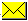# Standards for Astronomical Catalogues, Version 2.0 (pdf)(February 2000)

## 3.2  Standard of Catalogues: Units

The unit in which physical parameters are expressed is a fundamental parameter which becomes especially important when data are to be compared, or used outside a specialized field of science — most physicists have never heard of astronomy-specific units.

Special care has been taken to try to use the standard SI units, and to convert if necessary the unit to such standards: for instance, we use the string 0.1nm to express Angströms (Å=10–10m), since the Angström is a non-standard unit. Another example is mW/m2 , the milliwatt per m2, which is identical to the CGS erg/cm2/s unit, which is not used outside our discipline.

Only simple power functions of physical units are accepted, which means that e.g. solMass3/2 (solar mass at a 3/2 power) cannot be a valid unit. Some values are however traditionnally written in a decimal logarithmic scale, and we introduced bracketed units like [solMass] to indicate logM unit, i.e. a value representing solar masses expressed on a logarithmic scale.

The standard adopted here differs from the OGIP ones ( OGIP memo 93-001 about ``Specification of Physical Units within OGIP FITS files" by Ian M. George & Lorella Angelini, August 1993) for the syntax of composite units (operator symbols), and in the usage of math functions (only the square bracketted units, representing the log function is accepted here) and of obsolete CGS units which are not avoided here; the basic symbols however agree.

### 3.2.1  Syntax of Units

The syntax of the unit expression is summarized by the following rules:

Rule 1: any unit is described by a single word — no space is allowed. For instance, the Angström is coded 0.1nm, and never 0.1 nm; the kilometer-per-second is coded km/s, or km.s-1, but never km / s or km s-1.

Rule 2: the only allowed numerical factor is at the very beginning of the Unit string. The structure of the unit is therefore the concatenation of factor and unit_expression and we will write the ``number of pixels per Å" as 10pix/nm, and neither pix/0.1nm nor pix/(0.1nm).

The numerical factor may include the letter x for the multiplication, as 1.5x10+11 to express the number 1.5×1011

Rule 3: The operators to express a compound unit are

/ for the division — as in km/s . for the multiplication (the dot is however understood as a decimal point in the leading numerical factor) — as in kW.h nothing for a power — as m2 for m2 or 10+21 for 1021.
Note that + or signs are not operators, but represent the leading sign of numeric values.

Rule 4: a simple (non-compound) unit is made of a basic unit symbol, eventually preceded by a multiple prefix.

Among several possible expressions of a unit, it is preferable to choose the shortest one; this leads also to prefer the division (/) to the multiplication of the inverse, e.g. prefer km/s to km.s-1.

### 3.2.2  Basic symbols

Basic Unit Symbols

```
Symbol  Explanation  Definition
(c) —   Unitless value
(c) %   Unitless value, in percent	 10–2
(a) a  year (also yr)   365.25d = 31.5576×106s
(b) A	 Ampere
(a) AU  astronomical unit 1.49598×1011m
(a) arcmin  minute of arc 1/60 °
(a) arcsec  second of arc 1/60 arcmin
(e)	 barn	 barn (cross-section)  10–28 m2
(c)	 bit	 binary information unit (computer storage)
(c)	 byte	 byte (computer storage)  8 bit
C		 Coulomb (electric charge) 	A⋅s
(b) cd	 Candela (luminous intensity)
(c) ct	 Count (events)
D 	 Debye  (1/3)×10–29 C⋅m
(a) d 	 day 				 24h = 86.4×103s
(a) deg   degree of arc (°)  π/180 rad
(e) eV	 electron-Volt  1.602177×10–19 J
(b) g gram	 10–3 kg
(a) h  hour of time (sideral if appropriate)  3600s
H		 Henry (inductance)		 Wb/A
Hz	 Hertz	(frequency)  s–1
J		 Joule (energy)    		N⋅m
(a) Jy   Jansky  10–26 W/m2/Hz
(b) K	 Kelvin
lm	 lumen (luminous flux)		 cd⋅sr
lx	 lux (illuminance)		 lm/m2
(b) m	 metre
(a) mag  magnitudes
(a) mas  millisecond of arc (π/6.48)×10–8 rad
(a) min  minute of time (sideral if appropriate)
(b) mol	 mole
N		 Newton (force)     kg⋅m/s2
Ohm 	 (Ω) Ohm (electric resistance)	 V/A
Pa	 Pascal (pressure)  N/m2
(a) pc  parsec  3.0857 ×1016m
(c) pix  pixel (image element)
(e) Ry	 Rydberg (energy)
(1/2) (2πe2/hc)2mec2 = 13.60583 eV
(b) s	 second of time
S		 Siemens (electric conductance) 	A/V
(c) solLum    Solar luminosity  	3.826×1026 W
(c) solMass   Solar mass  	1.989×1030 kg
(c) Sun   Unit referring to the Sun (e.g. abundances)
T		 Tesla	(magnetic field intensity)  Wb/m2
V		 Volts (electric potential) 		W/A
W		 Watt (power)      		J/s
Wb	 Weber (magnetic flux)		 V⋅s
(c) yr  year (also a)   365.25d = 31.5576×106s
```
The basic symbols listed include basic standard SI units (b), the extensions listed by the IAU style book marked (a), other frequent physical extensions (e), and a few further extensions used at CDS (c).

### 3.2.3  Multiples

Symbols used to express multiples and submultiples

```
Symbol  Explanation  Value
d	 deci	 10–1
c	 centi	 10–2
m	 milli	 10–3
u	 micro (µ)	 10–6
n	 nano	 10–9
p	 pico	 10–12
f	 femto	 10–15
a	 atto	 10–18
z	 zepto	 10–21
y	 yocto	 10–24

Symbol  Explanation  Value
da	 deca	 10
h	 hecto	 102
k	 kilo	 103
M	 mega  106
G	 giga	 109
T	 tera	 1012
P	 peta	 1015
E	 exa    1018
Z	 zetta    1021
Y	 yotta    1024

```
The standard SI multiple and submultiple prefixes can be used; these are summarized in multiple. Note that a single prefix can only be attached to a unit symbol, which means that e.g. mmas must not be used to designate a µ-arc-second, but rather uarcsec.

See the Unit Calculator

©UDS/CNRS  Contact: